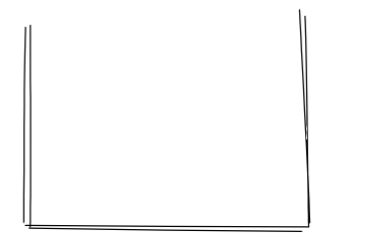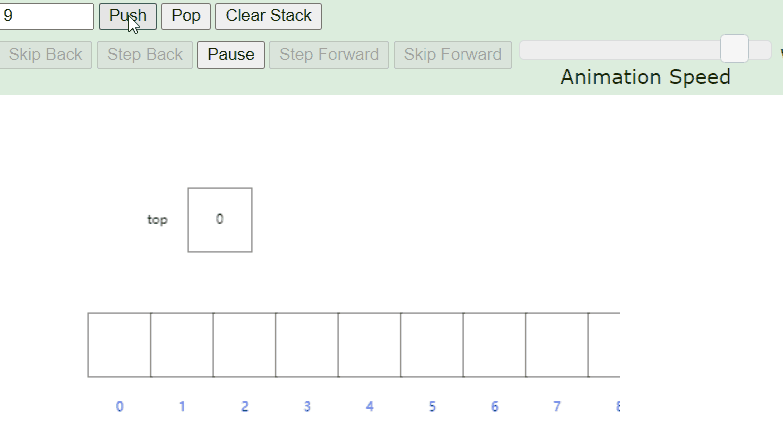📢 大家好，我是小丞同学，一名大二的前端爱好者

📢 这篇文章将讲解数据结构中的栈

📢 非常感谢你的阅读，不对的地方欢迎指正 🙏

📢 愿你忠于自己，热爱生活

## # 💡 内容抢先看

• 什么是栈？
• 栈结构有哪些方法
• 实现一个栈
• `LeetCode` 实战

📢 碎碎念

## # 一、什么是栈结构？`JS` 中，熟悉的执行上下文也使用栈结构来维护的，栈底是全局作用域（GO），当前执行代码的执行上下文依次加入栈中，栈顶的元素永远是正在执行的上下文对象

## # 二、栈结构有哪些方法呢？

`push()` 添加一个新元素到栈顶
`pop()` 移除栈顶元素，同时返回被移除的元素
`peek()` 返回栈顶元素，不改变栈
`isEmpty()` 判断栈是否为空
`clear()` 移除栈中所有元素
`size()` 返回栈中的元素个数

👇 👇 👇

## # 三、手写实现一个栈结构

### # 1. 创建一个 Stack 类

``````class Stack {
constructor() {
this.stackData = [];
}
}
``````
1
2
3
4
5

### # 2. 实现 push 方法

``````push(element) {
this.stackData.push(element)
}
``````
1
2
3

### # 3. 实现 pop 方法

`pop` 方法：删除数组的最后一位，并返回删除的值

``````pop() {
this.stackData.pop()
}
``````
1
2
3

``````const stack = new Stack()
stack.push(9)
stack.push(4)
stack.pop()
stack.push(6)
``````
1
2
3
4
5### # 4. 实现 peek 方法

`peek` 是查看栈顶的元素，也就是数组的最后一个元素，同时这个操作不会改变栈噢~只是查看

``````peek() {
return this.stackData[this.stackData.length - 1]
}
``````
1
2
3

``````const stack = new Stack()
stack.push(9)
stack.peek() // 9
``````
1
2
3

### # 5. 实现 size 方法

`size` 方法：返回栈中的元素个数，也就是返回数组的长度

``````size() {
return this.stackData.length
}
``````
1
2
3

``````const stack = new Stack()
stack.push(9)
stack.push(4)
stack.size() // 2
``````
1
2
3
4

### # 6. 实现 isEmpty 方法

`isEmpty` 方法：查看当前栈中是否有值，是空返回 `true`

``````isEmpty() {
return !this.stackData.length
}
``````
1
2
3

``````const stack = new Stack()
stack.push(9)
stack.isEmpty()  // false  不为空
``````
1
2
3

### # 7. 实现 clear 方法

`clear` 方法：清空栈，也就是重置一下栈

``````clear() {
this.stackData = []
}
``````
1
2
3

### # 8. 完整的栈结构

``````class Stack {
constructor() {
this.stackData = []
}
// 入栈
push(element) {
this.stackData.push(element)
}
// 出栈
pop() {
this.stackData.pop()
}
// 获取栈顶
peek() {
return this.stackData[this.stackData.length - 1]
}
// 检查是否为空
isEmpty() {
return !this.stackData.length
}
// 清空栈
clear() {
this.stackData = []
}
// 返回元素个数
size() {
return this.stackData.length
}
}
``````
1
2
3
4
5
6
7
8
9
10
11
12
13
14
15
16
17
18
19
20
21
22
23
24
25
26
27
28
29

## # 四、LeetCode 实战

#### # 20. 有效的括号 (opens new window)

• 当我们遇到左括号时，就把这个括号入栈
• 遇到右括号时，我们需要判断一下当前的栈顶是不是和这个括号匹配
• 如果匹配则说明符合，继续遍历，不匹配则直接返回 `false`
• 同时还有一种特殊情况，当输入的字符串 `s` 的长度是奇数时，不可能满足题意

``````var isValid = function (s) {
// 新建一个栈
const stack = [];
// 扫描字符串，遇到左括号入栈，遇到和栈顶括号类型匹配的右括号就出栈，类型不匹配直接 false
for (let i = 0; i < s.length; i++) {
// 如果为奇数直接弹出false
if (s.length % 2 === 1) {
return false
}
const c = s[i];
// 遇到左括号push
if (c === '(' || c === '{' || c === '[') {
stack.push(c)
} else {
const t = stack[stack.length - 1]
if (
(t === '(' && c === ')')||
(t === '[' && c === ']')||
(t === '{' && c === '}')
) {
stack.pop()
} else {
return false
}

};
}
return stack.length === 0
}
``````
1
2
3
4
5
6
7
8
9
10
11
12
13
14
15
16
17
18
19
20
21
22
23
24
25
26
27
28
29

## # 📖 总结

1. 利用数组封装了一个栈结构
2. 了解了栈结构的基本方法
3. 对数据结构有了进一步的了解

💌 如果文章有什么错误的地方，或者有什么疑问，欢迎留言，也欢迎私信交流

lastUpdate: 5/22/2022, 4:34:51 PM